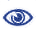# Prague Stringology Conference 2016

Computing Smallest and Largest Repetition Factorizations in O(n log n) Time

 Abstract: A factorization f1, . . ., fm of a string ,w is called a repetition factorization of w if each factor fi is a repetition, namely, fi = xkx' for some non-empty string x, an integer k ≥ 2, and x' being a proper prefix of x. Dumitran et al. (Proc. SPIRE 2015) proposed an algorithm which computes a repetition factorization of a given string w in O(n) time, where n is the length of w. In this paper, we propose two algorithms which compute smallest/largest repetition factorizations in O(n log n) time. The first algorithm is a simple O(n log n) space algorithm while the second one uses only O(n) space.

 Download paper:PostScript PDF BibTeX reference
 Download presentation: